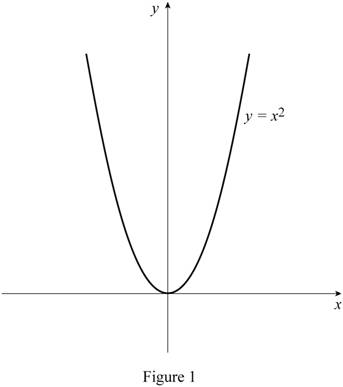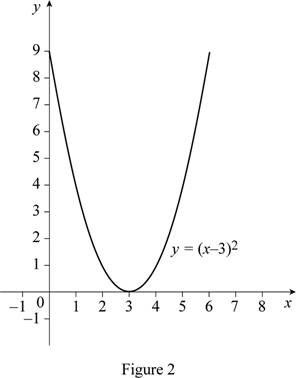# The function y = ( x − 3 ) 2 by hand without plotting any points.### Single Variable Calculus: Concepts...

4th Edition
James Stewart
Publisher: Cengage Learning
ISBN: 9781337687805### Single Variable Calculus: Concepts...

4th Edition
James Stewart
Publisher: Cengage Learning
ISBN: 9781337687805

#### Solutions

Chapter 1.3, Problem 10E
To determine

## To sketch: The function y=(x−3)2 by hand without plotting any points.

Expert Solution

### Explanation of Solution

The standard graph of the function y=x2 is roughly drawn as shown below in Figure 1.From Figure 1, it is observed that the curve y=x2 intersects the x-axis at the point (0,0).

Since the number 3 is subtracted from x in y=(x3)2, shift the graph y=x2 by 3 units to the right.

Thus, the graph of y=(x3)2 is shown below in Figure 2.From Figure 2, it is observed that the curve y=(x3)2 intersects the x-axis at the point (3,0).

### Have a homework question?

Subscribe to bartleby learn! Ask subject matter experts 30 homework questions each month. Plus, you’ll have access to millions of step-by-step textbook answers!